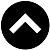-->

## MATHEMATICS FORM 4 SYLLABUS

1. Coordinate Geometry
1. Equation of a Line
1. Derive the general equation of a straight line
2. Midpoint of a Line Segment
1. Determine the coordinates of the midpoint of a line segment
3. Distance Between Two Points on a Plane
1. Calculate the distance between two points on a plane
4. Parallel and Perpendicular Lines
1. Compute gradients in order to determine the conditions for any two lines to be parallel
2. Compute gradients in order to determine the conditions for any two lines to be perpendicular
3. Solve problems on parallel and perpendicular lines
2. Area And Perimeter
1. Area of any Triangle
1. Derive the formula for the area of any triangle
2. Apply the formula to find the area of any triangle
2. Area of a Rhombus
1. Derive the formula for finding the area of rhombi in terms of the diagonals.
2. Apply the formula to find the areas of quadrilaterals
3. Perimeter of a Regular Polygon
1. Derive the formula for finding the length of a side of a regular polygon
2. The formula to determine the perimeter of a regular polygon
4. Area of a Regular Polygon
1. Derive the formula for finding the area of a regular polygon
2. Apply the formula to calculate the area of a regular polygon
5. Area of Similar Polygons
1. Find the ratio of areas of similar polygons
2. Solve problems related to ratio for areas of similar polygons
3. Three Dimensional Figures
1. Three Dimensional Figures
1. Classify three dimensional figures
2. List the characteristics of each class
2. Construction of Three Dimensional Figures
1. Construct three dimensional figures
3. Sketching Three Dimensional Figures
1. Sketch three dimensional figures
2. Identify properties of three dimensional figures
3. Find the angle between a line and a plane
4. Calculate the angle between two planes
4. Surface Area of Three Dimensional Objects
1. Derive the formulae for calculating the surface area of prisms, cylinder and pyramids and cone
2. Apply the formulae to calculate the surface area of spheres
5. Volume of Three Dimensional Objects
1. Derive the formulae for calculating volume of prisms, cylinders and pyramids
2. Apply the formulae to calculate the volume of cylinders, pyramids and cones
4. Probability
1. Probability of an Events
1. Determine the probability of an event through experiments
2. Interpret experimental results in relation to real life occurrences
3. Write the formula for finding the probability of an event
4. Apply the formula to calculate the probability of an event
2. Combined Events
1. Perform experiments of two combined events
2. Draw a tree diagram of combined events
3. Find the probability of two combined events using the formula
4. Apply the knowledge of probability to determine the occurrence of events in real life situation
5. Trignometry
1. Trigonometric Ratios
1. Determine the sine, cosine and tangent of an angle measured in the clockwise and anticlockwise directions
2. Apply trigonometric ratios to solve problems in daily life
2. Sine and Cosine Functions
1. Find sines and cosines of angles 0 such that -720°≤ᶿ≥ 720°
2. Draw the graphs of sine and cosine
3. Interpret the graphs of sine and cosine functions
3. Sine and Cosine Rules
1. Derive the sine and cosine rules
2. Apply the sine and cosine rules in solving problems on triangles
4. Compound Angles
1. Apply the compound of angle formulae or sine, cosine and tangent in solving trigonometric problems
6. Vectors
1. Displacement and Positions of Vectors
1. Explain the concept of a vector quantity
2. Distinguish between displacement and position vectors
3. Resolving any vector into I and J components
2. Magnitude and Direction of a Vector
1. Calculate the magnitude and direction of a vector
3. Sum and Difference of Vectors
1. Find the sum of two or more vectors
2. Find the difference of vectors
4. Multiplication of a Vector by a Scalar
1. Multiply a vector by a scalar
5. Application of Vectors
1. Apply vectors in solving simple problems on velocities, displacements and forces
7. Matrices And Transformations
1. Operations on Matrices
1. Explain the concept of a matrix
2. Add matrices of order up to 2 X 2
3. Subtract matrices of order up to 2 X 2
4. Multiply a matrix of order 2 X 2 by a scalar
5. Multiply two matrices of order up to 2 X 2
2. Inverse of a Matrix
1. Calculate the determinant of a 2 X 2 matrix
2. Find the inverse of a 2 X 2 matrix
3. Apply 2 X 2 matrix to solve simultaneous equations
3. Matrices and Transformations
1. Transform any point P(X, Y) into P¹(X¹,Y¹) by pre-multiplying (ᵡᵧ) with a transformation matrix T
2. Apply the matrix to reflect a point P(X, Y ) in the x-axis
3. Apply the matrix to reflect a point P(X, Y) in the Y-Axis
4. Use a matrix operator to rotate any point P( X, Y ) through 90° 180°, 270° and 360° about the Origin
5. Use the enlargement matrix E in enlarging figures
8. Linear Programming
1. Simultaneous Equations
1. Form simultaneous equation from word problems
2. Solve simultaneous equations graphically
2. Inequalities
1. Form linear inequalities in two unknowns from word problems
2. Find the solution set of simultaneous linear inequalities graphically
3. The Objective Function
1. Form an objective function from word problems
4. Maximum and Minimum Values
1. Locate corner points on the feasible region
2. Find the minimum and maximum values using the objective function